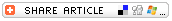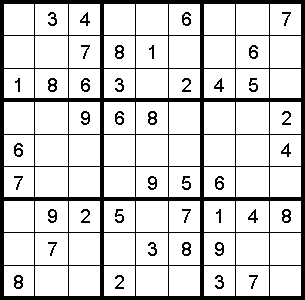## Search form

Home > Lesson Planning Channel > Lesson Planning Archives > Sudoku Puzzle Archive > Sudoku Puzzle Teaching Tips

SUDOKU PUZZLE

#Sudoku Puzzle -- Teaching TipsSudoku puzzles are an excellent tool for building students' critical thinking skills. It also builds confidence in their abilities to complete challenging tasks, which can carry over into many aspects of classroom performance.

Working Sudoku puzzles is a skill that most fourth graders can learn. It would be a good challenge activity for advanced third graders or to teach near the end of third grade.

In a Sudoku puzzle, students employ logic and the process of elimination to figure out the numbers that fit in each blank square of the puzzle grid. Each puzzle is "built" on a 27-square grid. Some numbers are provided. Students must use those numbers to help them determine the number that fits in each of the empty squares. Each Row, each Column, and each Block of 9 squares in the completed Sudoku puzzle must contain each of the numbers 1 to 9.

If your students are not familiar with how a Sudoku puzzle works, you will want to copy one of the puzzles onto a transparency or draw it out on a board or chart to use it as a teaching tool. For the purposes of this explanation, we will use this sample puzzle.Provide students with a copy of this Sudoku Puzzle (this is Puzzle #1 in the series) to work on as you walk through teaching some steps students might use as they complete it.

Point out to students that each puzzle contains

• 9 Rows of squares,
• 9 Columns of squares, and
• 9 Blocks, each made up of 9 squares.

For purposes of solving the puzzle, share with students that you will name

• the Rows from Row 1 to Row 9;
• the Columns from Column 1 to Column 9; and
• the Blocks from Block A to Block I in this manner:
--- Block A is the block in the upper left-hand corner of the grid, which comprises squares in Rows 1, 2, and 3 and Columns 1, 2, and 3;
--- Block B is the block in upper center that comprises squares in Rows 1, 2, and 3 and Columns 4, 5, and 6;
--- Block C is the block in upper right that comprises squares in Rows 1, 2, and 3 and Columns 7, 8, and 9;
--- Block D is the block in left-center of the grid, which comprises squares in Rows 4, 5, and 6 and Columns 1, 2, and 3

Next, introduce to students the concepts of elimination and narrowing down the possibilities.

A good strategy for beginning to solve a Suduko puzzle is to search for a Row or Column or Block that has many of its squares already filled in. In the sample puzzle above, Row 3 might be a good place to start because seven of the nine numbers in Row 3 are already provided. The numbers 1, 8, 6, 3, 2, 4, and 5 are provided, so you will want to model for students a thought processes that goes something like this:

Which of the numbers 1 to 9 are missing from Row 3 of the puzzle?

The numbers 7 and 9 are the only two numbers that are missing from Row 3.

The next step will be to determine if either of those two numbers appears in the Columns or Blocks that include the numbers in Row 3. The missing-number squares in Row 3 are also located in
--- Columns 5 and 9.
--- Block B.

So let's begin by looking in Column 5. Are either of the numbers 7 or 9 already provided in Column 5? Yes, the number 9 is already provided in Column 5, so the only possible number that could fit in Row 3, Column 5 is 7. You will write the number 7 in the empty square at Row 3, Column 5. To prove you have done this correctly, you might double-check yourself by verifying that no other 7 appears in
--- Row 3.
--- Column 5.
--- Block B.

Now the only other number not used in Row 3 is the number 9. And the only empty square in Row 3 is the square in Column 9. So you will want to model the thought process:

Is the number 9 already provided in Column 9? No, it is not, so 9 is the only possible number that could fit in Row 3, Column 9. So you will write the number 9 in the empty square at Row 3, Column 9. You might double-check by verifying that there is no other 9 in
--- Row 3.
--- Column 9.
--- Block C.

So now, students have filled in the entire length of Row 3 with the numbers 1, 8, 6, 3, 7, 2, 4, 5, and 9.

Students will continue in this manner. A next good move might be to look at Row 7. Like in Row 3, seven of the nine numbers are already provided in Row 7. The numbers 9, 2, 5, 7, 1, 4, and 8 are already provided. The only missing numbers are 3 and 6. Model the thought processes students might use as they try to figure out the best place to write those two numbers in Row 7:

The two empty squares in Row 7 are found in Columns 1 and 5. In order to determine the number that might fit in Row 7, Column 1 you should look at what numbers are already provided in that Column. Does Column 1 contain either of the two missing numbers? Yes, Column 1 already has in it the number 6, so the only possible number that will fit in Row 7, Column 1 is the number 3. You will write the number 3 in the empty square at Row 7, Column 1. You can double-check your work by verifying that there is no other 3 in
--- Row 7.
--- Column 1.
--- Block H.

That leaves only one remaining number, 6, which should fit in Row 7, Column 5. Is there a 6 in Column 5? No there is not, so you can write the number 6 in the empty square in Row 7, Column 5. You can double-check your work by verifying that there is no other 6 in
--- Row 7. --- Column 5. --- Block H.

Students will continue in this manner. Ask them to identify what a good next move might be. Students might say they would next look at Block B. Since they filled in the number 7 in an earlier step, now six of the nine numbers are provided in Block B. (Note: There are other good next moves. For example, six of nine numbers are now known in Column 5 and Block H too.)

When students look at Block B, their thought processes might go something like this:

• I know that the numbers 1, 2, 3, 6, 7, and 8 have already been used in Block B.

• The only numbers missing from Block B are 4, 5, and 9. In Block B,
--- Row 1 already has the number 4 in it, so the only possibilities for Row 1, Column 4 are 5 or 9. But if I look down Column 4, I see the number 5 has already been used there. So the only number that might fit in Row 1, Column 4 is the number 9. So I write the number 9 in the empty square at Row 1, Column 4. --- Since I have now used the number 9 in Block B, now I only need to place the missing numbers 4 and 5 --- Row 2 has neither of those numbers 4 or 5. But if I look down Column 6, I see the number 5 already appears in that Column. The only number that might fit in Row 2, Column 6 is 4, so I write the number 4 in the empty square at Row 2, Column 6. --- That leaves only one blank square in Block B. The only possibility for that empty square is the one remaining number, 5. So I write the number 5 in the blank square at Row 1, Column 5. --- I can double-check my work at this point by verifying that no number is repeated in Block B or in any of the Rows or Columns that are part of Block B.

Pose to students the question What would be the next good move to make? The best move at this point might be to try to figure out the missing numbers in Column 5 because seven or the nine numbers are already provided

At this point you might let students try to figure out the missing numbers in Column 5. The two missing numbers are 2 and 4. Which of those numbers fits in Column 5, Row 5? (The number 2 is the only one that fits there because there is already a 4in Row 5.) After writing the 2 in Row 5, Column 5, that leaves only one number, the 4, to fit into the square at Row 9, Column 5. That appears to be correct; I can verify that it is correct because there is no other 4 in Row 9, Column 5, or Block H

Like anything else, students will get more proficient in time as they do more Sudoku puzzles. Guide them through the first puzzle or two, and you will likely have students begging for more Sudokus. The best parts of all: students will be building confidence in their ability to tackle challenging tasks and critical thinking skills they are exercising will carry over into all aspects of their classroom performance.

Education World®